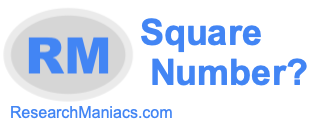Is 4 a square number?Here we will answer the question: "Is 4 a square number?" You could also ask "Is 4 a perfect square?" Square number and perfect square mean the same thing.

Anyway, for 4 to be a square number, 4 must be the product of an integer multiplied by itself. In other words, the square root of 4 must equal a whole number (integer).

The square root of 4 is 2 which is an integer. Thus, 4 is a square number.

The answer to the question: "Is 4 a square number?" is Yes.
The answer to the question: "Is 4 a perfect square?" is also Yes.

Square Number?
Enter another number to see if it is a square number:

Is 5 a square number?
Find out if the next number on our list is a perfect square.